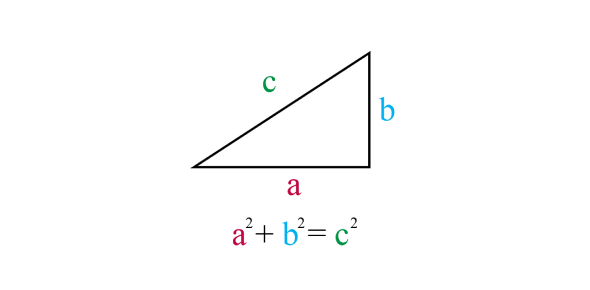How can Pythagorean Theorem be applied on spherical objects? - ProProfs DiscussTopicsMoreProductsMore+ Ask Question

# How can Pythagorean Theorem be applied on spherical objects?

Asked by K. Tanaka, Last updated: Feb 18, 2020

###Request 0FollowShareAnswer AnonymouslyAnswer LaterCopy Link#### John F. connor

Traveler, Avid Reader, Free as a bird

John F. connor, Content Marketing executive, MA, Minsk,PolandThe Pythagorean Theorem is known for calculating right triangle, but it also applies to spherical objects. It is hard to believe that the Pythagorean Theorem can be applied to spheres, but they can. The sphere has a right triangle in it. Then amounts of two sides have been given and then the hypotenuse or the other side can be determined.Then the rest of the circle and areas between the inner circle and the triangle can be determined based on formulas. Even though it sounds odd, the spherical triangle is actually two shapes together: the circle and the triangle within the circle. The law of cosine is sometimes applied to this. It could be cos (C/R is equal to the cosine of (A/R) times the cosine of (B/R).Search for Google imagesSelect a recommended image
Upload from your computerCancelSearch for Google imagesSelect a recommended image
Upload from your computerCancelSearch for Google imagesSelect a recommended image
Upload from your computerCancel